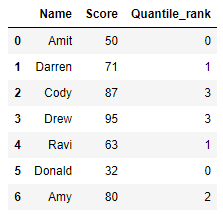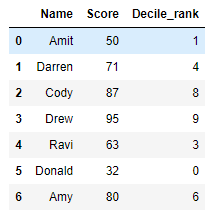# Quantile and Decile rank of a column in Pandas-Python

• Last Updated : 10 Jul, 2020

Let’s see how to find the Quantile and Decile ranks of a column in Pandas. We will be using the `qcut()` function of the `pandas` module.

## pandas.qcut()

Pandas library’s function `qcut()` is a Quantile-based discretization function. This means that it discretize the variables into equal-sized buckets based on rank or based on sample quantiles.

Syntax : pandas.qcut(x, q, labels=None, retbins: bool = False, precision: int = 3, duplicates: str = ‘raise’)

Parameters :

• x : 1d ndarray or Series.
• q : Number of quantiles. For example, 10 refers to deciles and 4 refers to quantiles.
• labels : Used as labels for the resulting bins. If it is set as False, it returns only integer indicators of the bins. If True, then it raises an error. By default, it is set to None.
• retbins : (Optional) It is a boolean which returns the (bins, labels) when set to True.
• precision : (Optional) The precision at which to store and display the bins labels.
• duplicates : (Optional) If bin edges are not unique, raise ValueError or drop non-uniques.

## Quantile Rank

Algorithm :

1. Import `pandas `and `numpy `modules.
2. Create a dataframe.
3. Use `pandas.qcut()` function, the `Score` column is passed, on which the quantile discretization is calculated. And `q` is set to 4 so the values are assigned from 0-3
4. Print the dataframe with the quantile rank.

 `# importing the modules``import` `pandas as pd``import` `numpy as np``   ` `# creating a DataFrame``df ``=` `{``'Name'` `: [``'Amit'``, ``'Darren'``, ``'Cody'``, ``'Drew'``,``                ``'Ravi'``, ``'Donald'``, ``'Amy'``],``      ``'Score'` `: [``50``, ``71``, ``87``, ``95``, ``63``, ``32``, ``80``]}``df ``=` `pd.DataFrame(df, columns ``=` `[``'Name'``, ``'Score'``])`` ` `# adding Quantile_rank column to the DataFrame``df[``'Quantile_rank'``] ``=` `pd.qcut(df[``'Score'``], ``4``,``                               ``labels ``=` `False``)`` ` `# printing the DataFrame``print``(df)`

Output :## Decile Rank

Algorithm :

1. Import `pandas `and `numpy `modules.
2. Create a dataframe.
3. Use `pandas.qcut()` function, the `Score` column is passed, on which the quantile discretization is calculated. And `q` is set to 10 so the values are assigned from 0-9
4. Print the dataframe with the decile rank.

 `# importing the modules``import` `pandas as pd``import` `numpy as np``   ` `# creating a DataFrame``df ``=` `{``'Name'` `: [``'Amit'``, ``'Darren'``, ``'Cody'``, ``'Drew'``,``                ``'Ravi'``, ``'Donald'``, ``'Amy'``],``      ``'Score'` `: [``50``, ``71``, ``87``, ``95``, ``63``, ``32``, ``80``]}``df ``=` `pd.DataFrame(df, columns ``=` `[``'Name'``, ``'Score'``])`` ` `# adding Decile_rank column to the DataFrame``df[``'Decile_rank'``] ``=` `pd.qcut(df[``'Score'``], ``10``,``                            ``labels ``=` `False``)`` ` `# printing the DataFrame``print``(df)`

Output :My Personal Notes arrow_drop_up